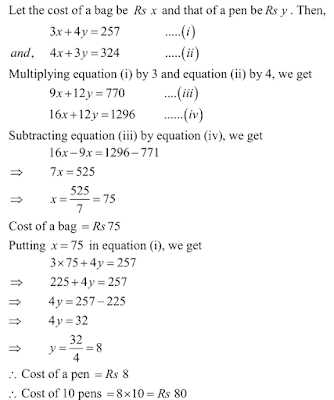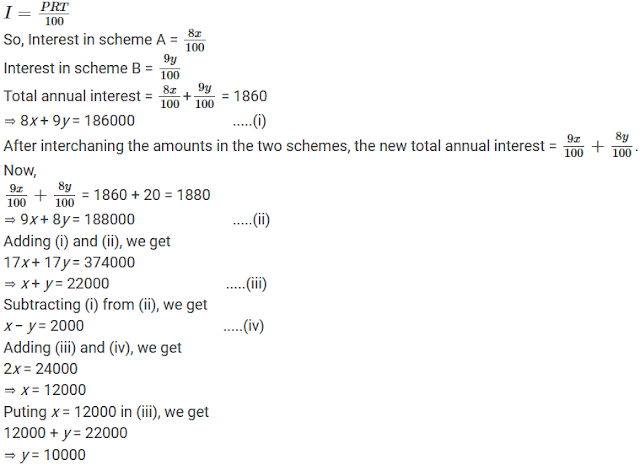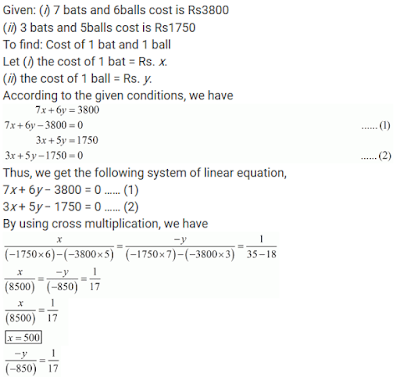## Chapter 3 Pair of Linear Equations in Two Variables R.D. Sharma Solutions for Class 10th Math Exercise 3.6

Exercise 3.6

In each of the following systems of equations determine whether the system has a unique solution, no solution or infinitely many solutions. In case there is a unique solution, find it:

1. 5 pens and 6 pencils together cost Rs 9 and 3 pens and 2 pencils cost Rs 5. Find the cost of1 pen and 1 pencil.

Solution2. 7 audio cassettes and 3 video cassettes cost Rs 1110, while 5 audio cassettes and 4 video cassettes cost Rs 1350. Find the cost of an audio cassette and a video cassette.

Solution

Let the cost of a audio cassette be Rs x and that of a video cassette be . Rs y Then,Hence, cost of one audio cassette = Rs 30 and cost of one video cassette = Rs 300 .

3. Reena has pens and pencils which together are 40 in number. If she has 5 more pencils and 5 less pens, then number of pencils would become 4 times the number of pens. Find the original number of pens and pencils.

Solution

Let the number of pens be x and that of pencil be y. then,4. 4 tables and 3 chairs, together, cost Rs 2,250 and 3 tables and 4 chairs cost Rs 1950. Find the cost of 2 chairs and 1 table.

Solution

Let the cost of a table be Rs x and that of a chairs be Rs y Then,Cost of 2 chairs = 300 Rs and cost of 1 table = 450
∴ The cost of 2 chairs and 1 table 300 + 450 + 750

5. 3 bags and 4 pens together cost Rs 257 whereas 4 bags and 3 pens together cost R 324. Find the total cost of 1 bag and 10 pens.

SolutionHence, the total cost of 1 bag and 10 pens 75+ 80 = 155.

6. 5 books and 7 pens together cost Rs 79 whereas 7 books and 5 pens together cost Rs 77. Find the total cost of 1 book and 2 pens.

Solution

Let the cost of a book be Rs x and that of a pen be Rs y. Then,Hence, the total cost of 1 book and 2 pens 6 + 14 = 20

7. Jamila sold a table and a chair for 1050 , thereby making a profit of 10% on a table and 25% on the chair . If she had taken a profit of 25% on the table and 10% on the chair she would have got Rs 1065. Find the cost price of each .

Solution

Let the CP of the table be Rs x and that of the chair be Rs y.8. Susan nvested certain amount of money in two shemes A and B, which offer interest at the rate of 8% per annum and 9% per annum, respectively . She received Rs 1860 as annual interest . However, had she interchanged the amount of investment in the two schemes, she would have received Rs 20 more as annual interest . How much money did she invest in each scheme ?

Solution

Let the money invested in Scheme A be Rs x and that in Scheme B be Rs y.So, the money invested in scheme A = Rs 12,000 and in scheme B = Rs 10,000.

9.The coach of a cricket team buys 7 bats and 6 balls for Rs 3800. Later, he buys 3 bats and 5 balls for Rs 1750. Find the cost of each bat and each ball.

Solutionx = 50
Hence, cost of 1 bat = x = 500
Hence, cost of 1 ball = x = 50

10. A lending library has a fixed charge for the first three days and additional charge for each day thereafter. Saritha paid Rs 27 for a book kept for seven days, while Susy paid Rs 21 for the book she kept for five days. Find the fixed charge and the charge for each extra day.

Solution

To find:
(1) the fixed charge
(2) The charge for each day

Let the fixed charge be Rs x
And the extra charge per day be Rs y.
According to the given conditions,
x + 4y = 27
x + 4y - 27 = 0 ...(i)
x + 2y = 21
x + 2y - 21 = 0 ...(ii)
Subtracting equation 1 and 2 we get
2y = 6
y = 3
Substituting the value of y in equation 1 we get
x + 4(3) - 27 = 0
x + 12 - 27 = 0
x - 15 = 0
x = 15
Hence the fixed charge is x = Rs 15 and the charge of each day y = Rs 3

11. The cost of 4 pens and 4 pencils boxes is 100 . Three times the cost of a pen is 15 more than the cost of a pencil box . Form the pair of linear equations for the above situation. Find the cost of a pen and a pencil box .

Solution

Let the cost of 1 pen be x and that of 1 pencil box be y.
Now,
Cost of 4 pens + Cost of 4 pencil boxes = Rs 100 (given)
⇒ 4x + 4y = 100
⇒ x + y = 25 ...(i)
Also,
3 × Cost of a pen = Cost of a pencil box + 15
3x = y + 15
⇒ 3x - y = 15 ...(ii)
Adding (i) and (ii), we get
4x = 40
⇒ x = 10
Putting x = 10 in (i), we get
10 + y = 25
⇒ y = 15
Thus, the cost of a pen = 10 and that of a pencil box = 15.

12. One says, "Give me a hundred, friend! I shall then become twice as rich as you." The other replies, "If you give me ten, I shall be six times as rich as you." Tell me what is the amount of their respective capital ?

Solution

To find:
(1) Total amount of A.
(2) Total amount of B.
Suppose A has Rs x and B has Rs y
According to the given conditions,
x + 100 = 2(y − 100)
x + 100 = 2y − 200
x − 2y = −300 ....(1)
and
y + 10 = 6(x − 10)
y + 10 = 6x − 60
6x − y = 70 ....(2)
Multiplying equation (2) by 2 we get
12x − 2y = 140 ....(3)
Subtracting (1) from (3), we get
11x = 440
x = 40
Substituting the value of x in equation (1), we get
40 - 2y = -300
-2y = -340
y = 170
Hence A has x = Rs40 and B has y = Rs170

13. A and B each have a certain number of mangoes. A says to B, "if you give 30 of your mangoes, I will have twice as many as left with you." B replies, "if you give me 10, I will have thrice as many as left with you." How many mangoes does each have?

Solution

To find:
(1) Total mangoes of A.
(2) Total mangoes of B.

Suppose A has x mangoes and B has y mangoes,
According to the given conditions,
x + 30 = 2(y-30)
x + 30 = 2y - 60
x - 2y + 30 + 60 = 0
x - 2y + 90 = 0
y + 10 = 3(x-10)
y + 0 = 3x - 30
y - 3x + 10 + 30 = 0
y - 3x + 40 = 0
Multiplying eq. 1 by (3),
3x + 6y + 270 = 0 .... (3) and
5y = 310
y = 310/5
y = 62
x - 2 × 62 + 90 = 0
x - 124 + 90 = 0
x - 34 = 0
x = 34
Hence A has 34 mangoes and B has 62 mangoes .

14. Vijay had some bananas, and he divided them into two lots A and B . He solds first lot at the rate of Rs 2 for 3 bananas and the second lot at the rate of Rs 1 per banana and got a total of 400 . If he had sold the first lot at the rate of 1 per banana and the second lot at the rate of Rs 4 per five bananas, his total collection would have been Rs 460 . Find the total number of bananas he had.

Solution

Let the bananas in lot A be x and that in lot B be y.
Vijay sold 3 bananas for Rs 2 in lot A.
So, the cost of x bananas in lot A = 2/3 x
∴ 2/3 x + y = 400
⇒ 2x + 32x + 3y = 1200n ...(i)
Now, if he sells the first lot at the rate of Rs 1 per banana and second for Rs 4 for 5 bananas, then
x + 4/5 y = Rs 460
⇒ 5x + 4y = 2300 ...(ii)
Solving (i) and (ii), we get
x = 300 and y = 200
So, the total number ob bananas = x+y = 300 + 200 = 500

15. On selling a T.V. at 5% gain and a fridge at 10% gain, a shopkeeper gains Rs 2000. But if he sells the T.V. at 10% gain the fridge at 5% loss. He gains Rs 1500 on the transaction. Find the actual prices of T.V. and fridge

Solution

Given:
(i) On selling of a T.V. at 5% gain and a fridge at 10% gain, shopkeeper gain Rs.2000.
(ii) Selling T.V. at 10% gain and fridge at 5% loss. He gains Rs. 1500.
To find: Actual price of T.V. and fridge.